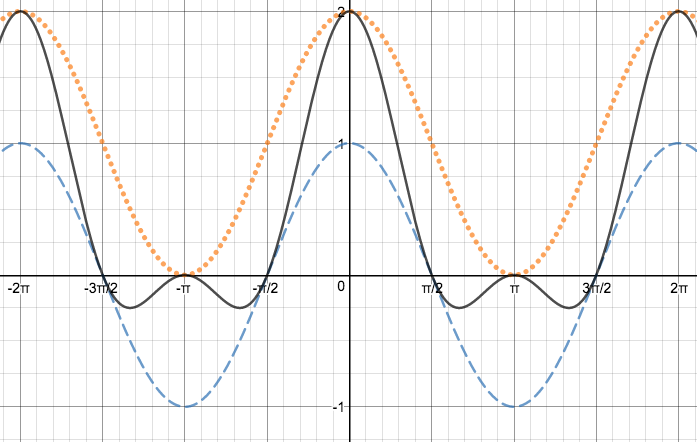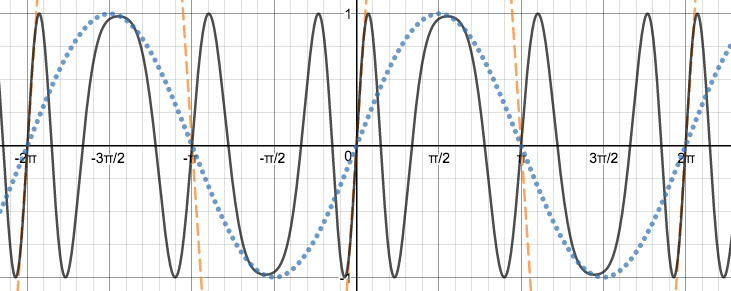## Modulation Synthesis

Have you ever wondered what AM and FM meant when scrolling through radio stations? AM stands for amplitude modulation and uses changes in amplitude to encode a radio wave. FM stands for frequency modulation which unlike AM, uses changes in frequencies to encode. The different techniques make a huge difference in terms of sound quality.

There are many different techniques we can use to digitally synthesize (create) sounds. Modulating a wave refers to changing its properties such as frequency, amplitude, and phase to create a new sound. For AM and FM synthesis, we will have two waves: a carrier wave and a modulator wave. The carrier wave is the original wave that we will be altering. The modulator wave refers to the wave that we will be using to alter the carrier wave. It’s more like the factor that changes the carrier wave. For this tutorial, we will be learning how to implement these techniques through very basic modulation.

## Amplitude modulation (AM) Synthesis

Amplitude Modulation involves having a carrier wave’s amplitude being driven by the modulator wave. It is often understood as the sum and differences between the signals.

#### Example Program

``````use Libraries.Compute.Math
use Libraries.Sound.Audio
use Libraries.Sound.AudioSamples
// AM Synthesis

Math math
number twoPi = math:pi * 2
number carrierFrequency = 440 // Frequency of Carrier wave
number modulatorFrequency = 10 // Frequency of Modulator wave
number carrierAmplitude = 1 // Amplitude of Carrier wave
number modulatorAmplitude = 1 // Amplitude of Modulator wave

AudioSamples amWave
amWave:SetChannels(1) // Mono sound
amWave:SetSizeInSeconds(4) // 4 Seconds of playback

number sampleSize = amWave:GetSamplesPerSecond()
integer counter = 0
repeat while counter < amWave:GetSize()
number carrierWave = math:Cosine(carrierFrequency * twoPi * counter/sampleSize) // Carrier Wave
number modulatorWave = modulatorAmplitude * math:Cosine(modulatorFrequency * twoPi * counter/sampleSize) + carrierAmplitude // Modulator Wave
number a = carrierWave * modulatorWave // AM modulated wave
amWave:Set(counter, a, 0)
counter = counter + 1
end

Audio audio
audio:PlayUntilDone()``````

This may seem a little confusing using multiple waves and trigonometry, but the structure is very similar to the structure we’ve been using with our previous wave generators. What we have is two waves, the carrier and modulator wave mentioned earlier, that are combined (multiplied).

``````number carrierWave = math:Cosine(carrierFrequency * twoPi * counter/sampleSize) // Carrier Wave
number modulatorWave = modulatorAmplitude * math:Cosine(modulatorFrequency * twoPi * counter/sampleSize) + carrierAmplitude // Modulator Wave
number a = carrierWave * modulatorWave // AM modulated wave
``````

Combining these two waves creates a single wave that has the same frequency as the carrier, but with different amplitudes throughout each cycle. This is the basic formula for AM synthesis. The image below shows the resulting wave which should resemble something beating.### Frequency Modulation (FM) Synthesis

Frequency modulation is more widely used in real-life technologies than amplitude modulation because of its ability to “block�? noises. This provides a cleaner, crisper sound that is ultimately less staticky. As the name implies, we are changing the frequency of the carrier wave here while we leave the amplitude and phase the same. To visualize FM waves, imagine a slinky. If you were to compress the slinky, the frequency increases but stretching it out would decrease its frequency. What we are doing is compressing the wave at certain points to produce a new sound.

#### Example Program

To understand FM synthesis in Quorum, let’s take a look at the following example program.
``````use Libraries.Compute.Math
use Libraries.Sound.Audio
use Libraries.Sound.AudioSamples
// FM Synthesis

Math math
number twoPi = math:pi * 2
number carrierFrequency = 440 // Frequency of Carrier wave
number modulatorFrequency = 10 // Frequency of Modulator wave
number carrierAmplitude = 1 // Amplitude of Carrier wave
number modulatorAmplitude = 10 // Amplitude of Modulator wave

AudioSamples fmWave
fmWave:SetChannels(1) // Mono sound
fmWave:SetSizeInSeconds(4) // 4 Seconds of playback

number sampleSize = fmWave:GetSamplesPerSecond()
integer counter = 0
repeat while counter < fmWave:GetSize()
number modulatorWave = modulatorAmplitude * math:Sine(modulatorFrequency * twoPi * counter/sampleSize) // Modulator Wave
number f = carrierAmplitude * math:Sine(carrierFrequency * twoPi * counter/sampleSize + modulatorWave) // FM Modulated wave
fmWave:Set(counter, f, 0)
counter = counter + 1
end

Audio audio
audio:PlayUntilDone()``````

Again we alter the carrier wave with the modulator wave. The equations are different from the equations used for AM synthesis. What we have is a carrier wave that includes another wave (modulator wave) into itself to change its sound.

``````number modulatorWave = modulatorAmplitude * math:Sine(modulatorFrequency * twoPi * counter/sampleSize) // Modulator Wave
number f = carrierAmplitude * math:Sine(carrierFrequency * twoPi * counter/sampleSize + modulatorWave) // FM Modulated wave
``````

The image below shows the waves being combined to alter the carrier wave’s frequencies. This is the most basic form of FM synthesis. The sound produced should sound similar to quickly placing and removing your hand from a speaker’s output.## Next Tutorial

In the next tutorial, we will discuss Mixing Signals, which describes how to mix different sounds together..